Anúncio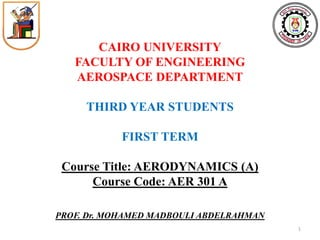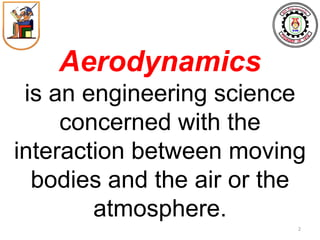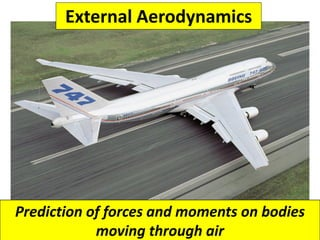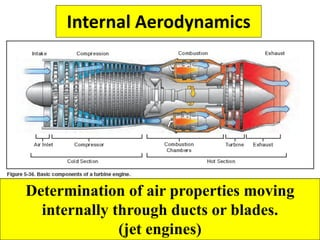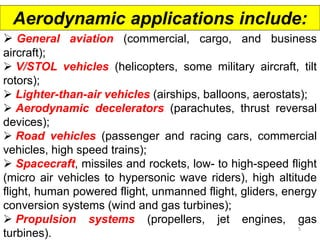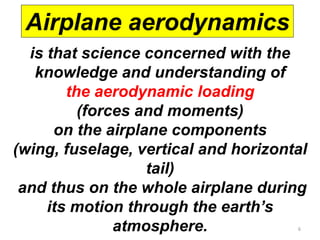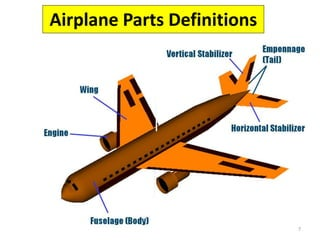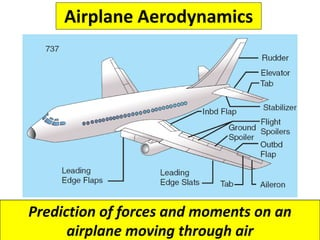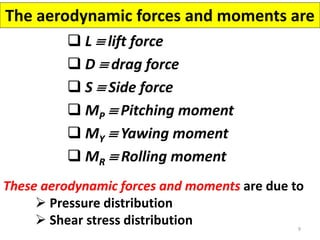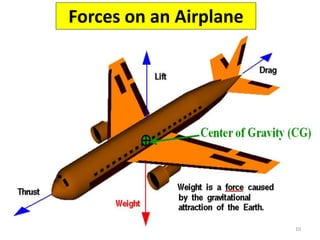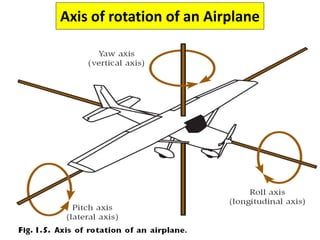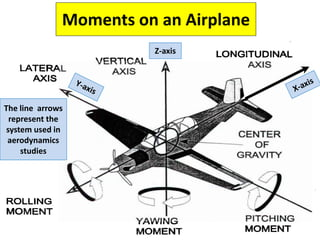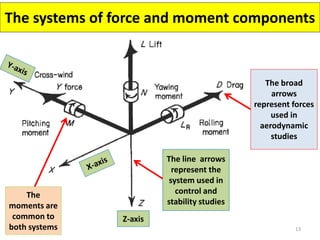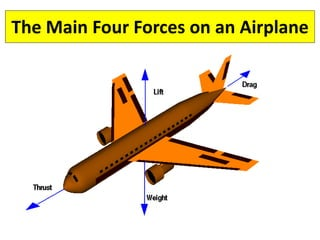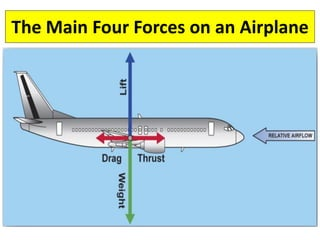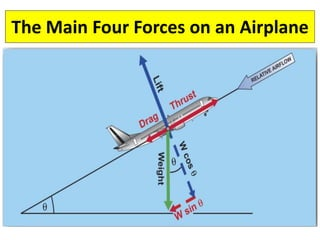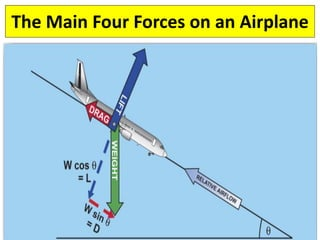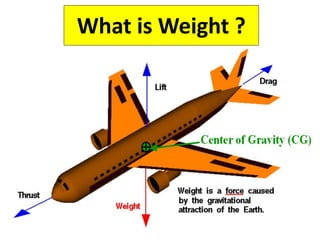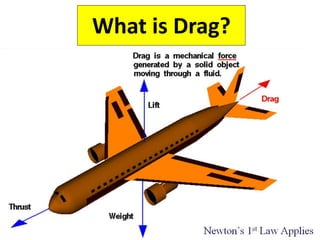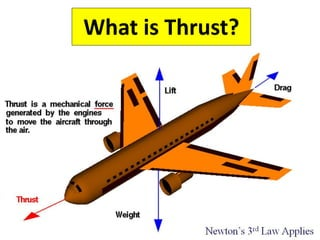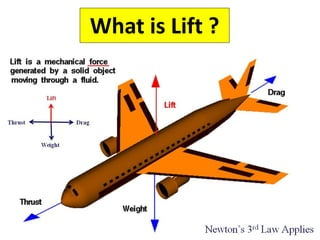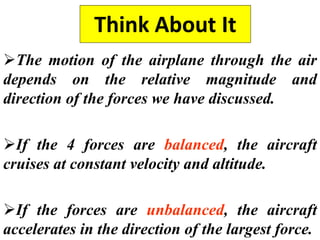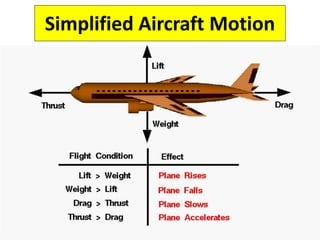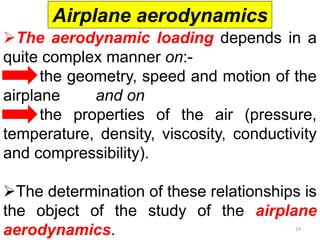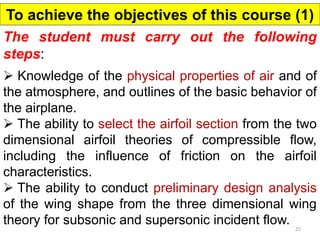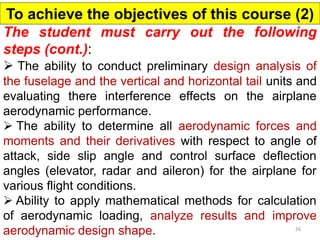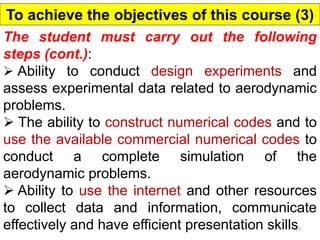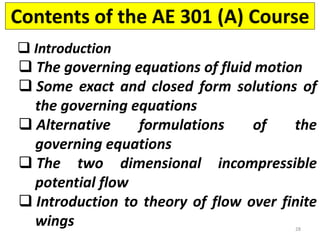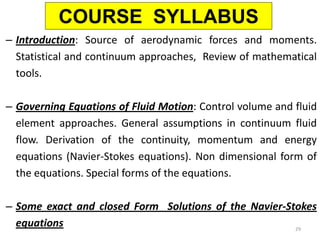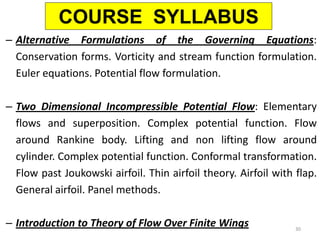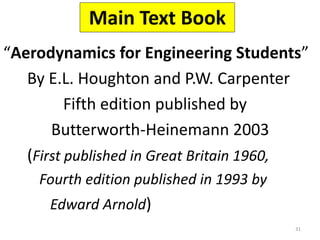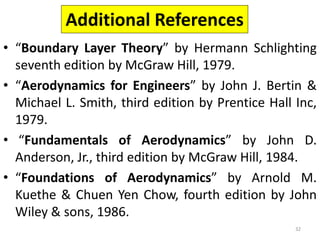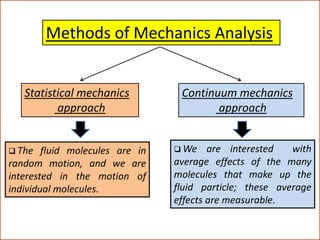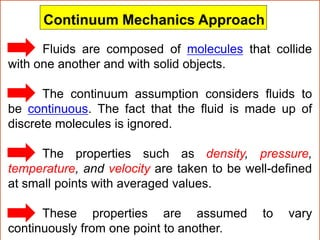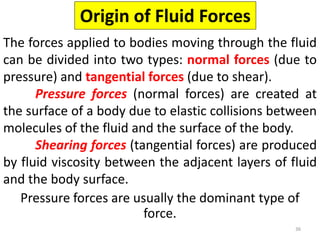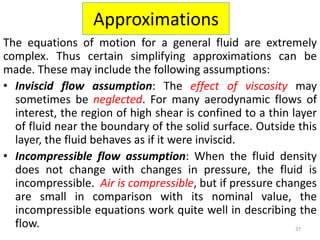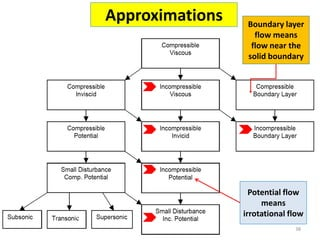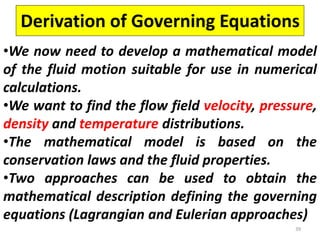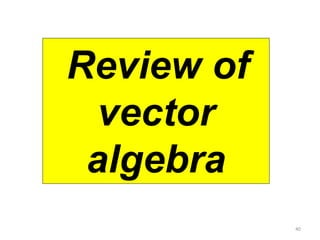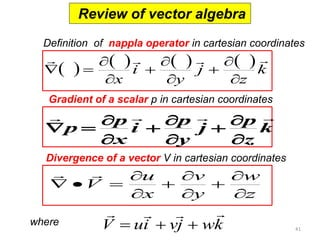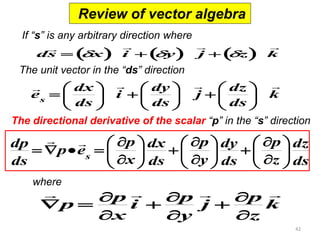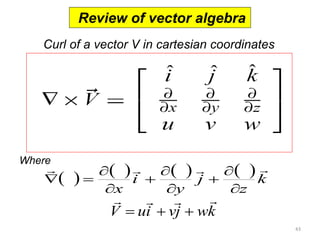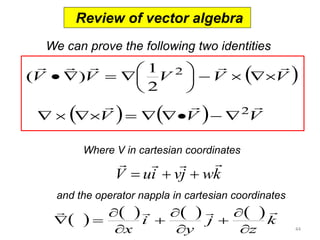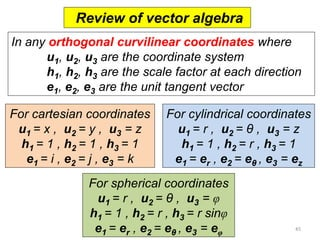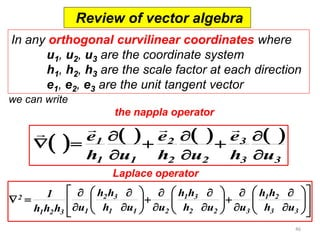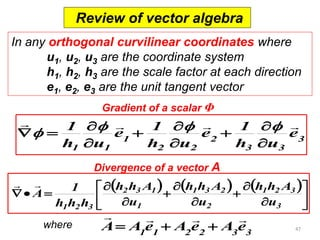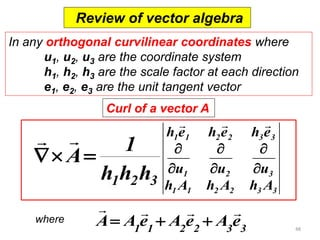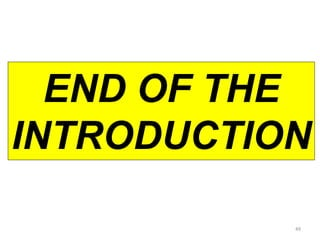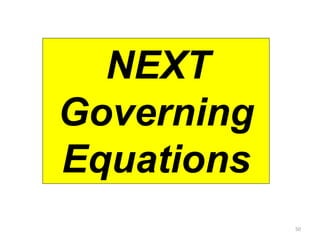1 de 50
Anúncio

### 1. introduction aerodynamics

1. CAIRO UNIVERSITY FACULTY OF ENGINEERING AEROSPACE DEPARTMENT THIRD YEAR STUDENTS FIRST TERM Course Title: AERODYNAMICS (A) Course Code: AER 301 A PROF. Dr. MOHAMED MADBOULI ABDELRAHMAN 1
2. Aerodynamics is an engineering science concerned with the interaction between moving bodies and the air or the atmosphere. 2
3. 10/14/2011 3 COURSE OBJECTIVES External Aerodynamics External Aerodynamics Prediction of forces and moments on bodies moving through air
4. Internal Aerodynamics 4 Determination of air properties moving internally through ducts or blades. (jet engines)
5. Aerodynamic applications include:  General aviation (commercial, cargo, and business aircraft);  V/STOL vehicles (helicopters, some military aircraft, tilt rotors);  Lighter-than-air vehicles (airships, balloons, aerostats);  Aerodynamic decelerators (parachutes, thrust reversal devices);  Road vehicles (passenger and racing cars, commercial vehicles, high speed trains);  Spacecraft, missiles and rockets, low- to high-speed flight (micro air vehicles to hypersonic wave riders), high altitude flight, human powered flight, unmanned flight, gliders, energy conversion systems (wind and gas turbines);  Propulsion systems (propellers, jet engines, gas turbines). 5
6. is that science concerned with the knowledge and understanding of the aerodynamic loading (forces and moments) on the airplane components (wing, fuselage, vertical and horizontal tail) and thus on the whole airplane during its motion through the earth’s atmosphere. Airplane aerodynamics 6
7. Airplane Parts Definitions 7
8. Airplane Aerodynamics 8 Prediction of forces and moments on an airplane moving through air
9. The aerodynamic forces and moments are  L  lift force  D  drag force  S  Side force  MP  Pitching moment  MY  Yawing moment  MR  Rolling moment These aerodynamic forces and moments are due to  Pressure distribution  Shear stress distribution 9
10. Forces on an Airplane 10
11. 11 Axis of rotation of an Airplane
12. 12 Moments on an Airplane The line arrows represent the system used in aerodynamics studies Z-axis
13. 13 The systems of force and moment components The broad arrows represent forces used in aerodynamic studies The line arrows represent the system used in control and stability studies The moments are common to both systems Z-axis
14. The Main Four Forces on an Airplane
15. The Main Four Forces on an Airplane
16. The Main Four Forces on an Airplane
17. The Main Four Forces on an Airplane
18. What is Weight ?
19. What is Drag?
20. What is Thrust?
21. What is Lift ?
22. Think About It The motion of the airplane through the air depends on the relative magnitude and direction of the forces we have discussed. If the 4 forces are balanced, the aircraft cruises at constant velocity and altitude. If the forces are unbalanced, the aircraft accelerates in the direction of the largest force.
23. Simplified Aircraft Motion
24. The aerodynamic loading depends in a quite complex manner on:-  the geometry, speed and motion of the airplane and on  the properties of the air (pressure, temperature, density, viscosity, conductivity and compressibility). The determination of these relationships is the object of the study of the airplane aerodynamics. Airplane aerodynamics 24
25. The student must carry out the following steps:  Knowledge of the physical properties of air and of the atmosphere, and outlines of the basic behavior of the airplane.  The ability to select the airfoil section from the two dimensional airfoil theories of compressible flow, including the influence of friction on the airfoil characteristics.  The ability to conduct preliminary design analysis of the wing shape from the three dimensional wing theory for subsonic and supersonic incident flow. To achieve the objectives of this course (1) 25
26. The student must carry out the following steps (cont.):  The ability to conduct preliminary design analysis of the fuselage and the vertical and horizontal tail units and evaluating there interference effects on the airplane aerodynamic performance.  The ability to determine all aerodynamic forces and moments and their derivatives with respect to angle of attack, side slip angle and control surface deflection angles (elevator, radar and aileron) for the airplane for various flight conditions.  Ability to apply mathematical methods for calculation of aerodynamic loading, analyze results and improve aerodynamic design shape. To achieve the objectives of this course (2) 26
27. The student must carry out the following steps (cont.):  Ability to conduct design experiments and assess experimental data related to aerodynamic problems.  The ability to construct numerical codes and to use the available commercial numerical codes to conduct a complete simulation of the aerodynamic problems.  Ability to use the internet and other resources to collect data and information, communicate effectively and have efficient presentation skills To achieve the objectives of this course (3) 27
28. Contents of the AE 301 (A) Course  Introduction  The governing equations of fluid motion  Some exact and closed form solutions of the governing equations  Alternative formulations of the governing equations  The two dimensional incompressible potential flow  Introduction to theory of flow over finite wings 28
29. –Introduction: Source of aerodynamic forces and moments. Statistical and continuum approaches, Review of mathematical tools. –Governing Equations of Fluid Motion: Control volume and fluid element approaches. General assumptions in continuum fluid flow. Derivation of the continuity, momentum and energy equations (Navier-Stokes equations). Non dimensional form of the equations. Special forms of the equations. –Some exact and closed Form Solutions of the Navier-Stokes equations COURSE SYLLABUS 29
30. –Alternative Formulations of the Governing Equations: Conservation forms. Vorticity and stream function formulation. Euler equations. Potential flow formulation. –Two Dimensional Incompressible Potential Flow: Elementary flows and superposition. Complex potential function. Flow around Rankine body. Lifting and non lifting flow around cylinder. Complex potential function. Conformal transformation. Flow past Joukowski airfoil. Thin airfoil theory. Airfoil with flap. General airfoil. Panel methods. –Introduction to Theory of Flow Over Finite Wings COURSE SYLLABUS 30
31. Main Text Book “Aerodynamics for Engineering Students” By E.L. Houghton and P.W. Carpenter Fifth edition published by Butterworth-Heinemann 2003 (First published in Great Britain 1960, Fourth edition published in 1993 by Edward Arnold) 31
32. Additional References •“Boundary Layer Theory” by Hermann Schlighting seventh edition by McGraw Hill, 1979. •“Aerodynamics for Engineers” by John J. Bertin & Michael L. Smith, third edition by Prentice Hall Inc, 1979. • “Fundamentals of Aerodynamics” by John D. Anderson, Jr., third edition by McGraw Hill, 1984. •“Foundations of Aerodynamics” by Arnold M. Kuethe & Chuen Yen Chow, fourth edition by John Wiley & sons, 1986. 32
33. 33 Fluid Mechanics Liquid (water, others) Gas (Air, others) Static Dynamic Aerodynamic is a branch of the fluid mechanics Aerodynamic
34. 34 Methods of Mechanics Analysis Statistical mechanics approach Continuum mechanics approach  The fluid molecules are in random motion, and we are interested in the motion of individual molecules.  We are interested with average effects of the many molecules that make up the fluid particle; these average effects are measurable.
35. 35 Fluids are composed of molecules that collide with one another and with solid objects. The continuum assumption considers fluids to be continuous. The fact that the fluid is made up of discrete molecules is ignored. The properties such as density, pressure, temperature, and velocity are taken to be well-defined at small points with averaged values. These properties are assumed to vary continuously from one point to another. Continuum Mechanics Approach
36. Origin of Fluid Forces The forces applied to bodies moving through the fluid can be divided into two types: normal forces (due to pressure) and tangential forces (due to shear). Pressure forces (normal forces) are created at the surface of a body due to elastic collisions between molecules of the fluid and the surface of the body. Shearing forces (tangential forces) are produced by fluid viscosity between the adjacent layers of fluid and the body surface. Pressure forces are usually the dominant type of force. 36
37. Approximations The equations of motion for a general fluid are extremely complex. Thus certain simplifying approximations can be made. These may include the following assumptions: •Inviscid flow assumption: The effect of viscosity may sometimes be neglected. For many aerodynamic flows of interest, the region of high shear is confined to a thin layer of fluid near the boundary of the solid surface. Outside this layer, the fluid behaves as if it were inviscid. •Incompressible flow assumption: When the fluid density does not change with changes in pressure, the fluid is incompressible. Air is compressible, but if pressure changes are small in comparison with its nominal value, the incompressible equations work quite well in describing the flow. 37
38. Approximations 38 Boundary layer flow means flow near the solid boundary Potential flow means irrotational flow
39. Derivation of Governing Equations •We now need to develop a mathematical model of the fluid motion suitable for use in numerical calculations. •We want to find the flow field velocity, pressure, density and temperature distributions. •The mathematical model is based on the conservation laws and the fluid properties. •Two approaches can be used to obtain the mathematical description defining the governing equations (Lagrangian and Eulerian approaches) 39
40. 40 Review of vector algebra
41. Definition of nappla operator in cartesian coordinates Gradient of a scalar p in cartesian coordinates Divergence of a vector V in cartesian coordinates where V ui vj wk        Review of vector algebra 41         k z j y i x               k z p j y p i x p p               z w y v x u V            
42. 42 Review of vector algebra ds  x i  y j  z k           If “s” is any arbitrary direction where k ds dz j ds dy i ds dx e s                                The unit vector in the “ds” direction The directional derivative of the scalar “p” in the “s” direction k z p j y p i x p p               where ds dz z p ds dy y p ds dx x p p e ds dp s                                     
43.         k z j y i x               Curl of a vector V in cartesian coordinates Curl of a vector Where V ui vj wk        Review of algebra 43
44. We can prove the following two identities Curl of a vector Where V in cartesian coordinates V ui vj wk        Review of vector algebra         k z j y i x               44 and the operator nappla in cartesian coordinates    V   V  V V V V V V         2 2 2 1 ( )                 
45. In any orthogonal curvilinear coordinates where u1, u2, u3 are the coordinate system h1, h2, h3 are the scale factor at each direction e1, e2, e3 are the unit tangent vector ReviCeuwrl oof fa vveecctotro r algebra 45 For cartesian coordinates u1 = x , u2 = y , u3 = z h1 = 1 , h2 = 1 , h3 = 1 e1 = i , e2 = j , e3 = k For cylindrical coordinates u1 = r , u2 = θ , u3 = z h1 = 1 , h2 = r , h3 = 1 e1 = er , e2 = eθ , e3 = ez For spherical coordinates u1 = r , u2 = θ , u3 = φ h1 = 1 , h2 = r , h3 = r sinφ e1 = er , e2 = eθ , e3 = eφ
46. Curl of a vector we can write the nappla operator Review of vector algebra 46 In any orthogonal curvilinear coordinates where u1, u2, u3 are the coordinate system h1, h2, h3 are the scale factor at each direction e1, e2, e3 are the unit tangent vector Laplace operator         3 3 3 2 2 2 1 1 1 h u e h u e h u e                                                               3 3 1 2 2 2 3 1 3 1 1 2 2 3 1 2 3 1 2 h u h h h u u h h h u u h h h h h u 1
47. ReviCeuwrl oof fa vveecctotro r algebra 47 Gradient of a scalar Φ In any orthogonal curvilinear coordinates where u1, u2, u3 are the coordinate system h1, h2, h3 are the scale factor at each direction e1, e2, e3 are the unit tangent vector Divergence of a vector A 1 1 2 2 3 3 A A e A e A e     where    3 3 3 2 2 2 1 1 1 e h u 1 e h u 1 e h u  1                                      3 1 2 3 2 1 3 2 1 2 3 1 1 2 3 u h h A u h h A u h h A h h h 1 A  
48. ReviCeuwrl oof fa vveecctotro r algebra 48 Curl of a vector A 1 1 2 2 3 3 A A e A e A e     where    In any orthogonal curvilinear coordinates where u1, u2, u3 are the coordinate system h1, h2, h3 are the scale factor at each direction e1, e2, e3 are the unit tangent vector 1 1 2 2 3 3 1 2 3 1 1 2 2 3 3 h A h A h A u u u h e h e h e 1 2 3 h h h 1 A             
49. 49 END OF THE INTRODUCTION
50. 50 NEXT Governing Equations
Anúncio